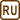## Deflection of the First Diffraction Maximum at a Grating

### Task number: 1974

Monochromatic light with a wavelength of 500 nm is falling normal onto an optical grating that has a groove period of 10 μm. Assess the angle α by which the first-order maximum is deflected from the normal.

• #### Hint 1

What is the meaning of the groove period?

• #### Hint 2

Find the path difference of rays shining from two adjacent slits onto a point on a far screen that is not located at the optical axis.

• #### Hint 3

What is the condition for two rays to produce an interference maximum?

• #### Notation

 b = 10−2 mm = 10−5 m Groove period λ = 500 nm = 5.0·10−7 m Wavelength of the diffracted light k = 1 Order of the maximum α = ? (°) Deflection angle from the normal
• #### Solution

A monochromatic light bends (diffracts) at individual slits of the grating and an interference pattern is formed on the screen. The position of interference maxima (bright fringes) and minima (dark fringes) on the screen depends on the path differences of the rays coming out of adjacent slits of the grating.

Let us presume that the screen is so far from the grating that the rays falling to a certain point P at the screen from different slits are approximately parallel.It is then true in the indicated right-angled triangle that:

$\mathrm{Δ}l = \,b\sin\,α,$

where Δl is the path difference between rays falling to point P from two adjacent slits, b is the groove period (the distance between two adjacent slits) and α is the angle between the rays and the normal to the grating.

We express the angle α and we get:

$α= \,\arcsin\, \frac{\mathrm{Δ}l}{b}.$

For a bright fringe (interference maximum) at point P, the path difference Δl must be:

$\mathrm{Δ}l = k λ,$

where k is the order number of the maximum; the assignment tells us to look for the first-order maxiumum, k = 1.

We substitute the third equation into the second one, use the given values and we get:

$α= \,\arcsin \,\frac{λ}{b}=\,\arcsin \,\frac{5.0{\cdot}10^{−7}}{1{\cdot}10^{−5}}=\,2°52'.$
• #### Answer

The first-order maximum is deflected by 2°51′ from the perpendicual to the grating.